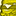# New to Qlik Sense

If you’re new to Qlik Sense, start with this Discussion Board and get up-to-speed quickly.

Announcements
Join us for a live Q&A! September 21, 10 AM ET - Onboarding Fast in Qlik Sense SaaS! REGISTER
cancel
Showing results for
Did you mean:Creator III

## Dynamically changing number formats?

I have some numbers with a very wide range of values, from \$10 to \$10,000,000,000+

At the moment I am using an expression which is SUM ([Invoice Value])/1000000 to get the values into "Millions of dollars" but for very large and very small numbers this looks ugly.

I can format the underlying number so if it is over a Million, it is divided by a Million, otherwise divide it by 1000;

( IF SUM ([Invoice Value]) > 1000000, SUM ([Invoice Value]) / 1000000 , SUM ([Invoice Value]) / 1000 )

But I cannot seem to work out the correct number-formatting codes, so if the number is over \$1M, it is shown with an M (\$320M), otherwise it is shown with a k (\$256k)

Just for reference, In Excel I would do; [>1000000]\$0.0,," M";[>1000]\$0.0,, "k";\$0 is there something similar in Qlik Sense?

16 RepliesMVP

Something like below

IF([Invoice Value] >= 1000000, [Invoice Value]/1000000 &'M',

If([Invoice Value] >= 1000, [Invoice Value]/1000 &'K', [Invoice Value]))MVP

Dual(

IF([Invoice Value] >= 1000000000, '\$'&[Invoice Value]/1000000000 & 'B',

IF([Invoice Value] >= 1000000, '\$'&[Invoice Value]/1000000 &'M',

If([Invoice Value] >= 1000, '\$'&[Invoice Value]/1000 &'K', '\$'&[Invoice Value]))),[Invoice Value])Creator III
Author

These are all valid formulas but they convert the values into strings so don't get plotted onto charts, etc.  These expressions don't work in the formatting of the results.Not applicable

You can also tweak with variable as well.Creator III
Author

Thanks, but I can't see how to do this with variables - are you able to explain (or write some code to demonstrate?)Not applicable

have you found the answer to this yet?MVP

I create a variable in the script like this:

SET vScaleNumber=if(\$1>1000000, num(\$1/1000000,'#,##0.000M')

,if(\$1>1000, num(\$1/1000,'#,##0.000K')

,num(\$1,'#,##0')

));

And then use it in expressions like this:

\$(vScaleNumber(sum(InvoiceValue)))

-Rob

http://qlikviewcookbook.comCreator III
Author

Oooh, thanks, that's very useful.

And you can extend that to currency format as well, I guess;

"If ([Sale Location] = 'USA', num(\$1,"\$0,0"), num(\$1,"£0.0"))Creator III
Author

Apologies for the long delay, but I just tried this in one of my dashboards, but it didn't work.

Should i be using the "expression" (\$(vScaleNumber(sum(InvoiceValue)))) in the script, or should it work in the measures of an object as well (that's where I'm trying to use it).

If I format the numbers in the script I will get text instead of numbers, so I shan't be able to plot the numbers on a chart, but if I add the measure to a chart it just gives me a blank.

<confused>Tags
Community Browser